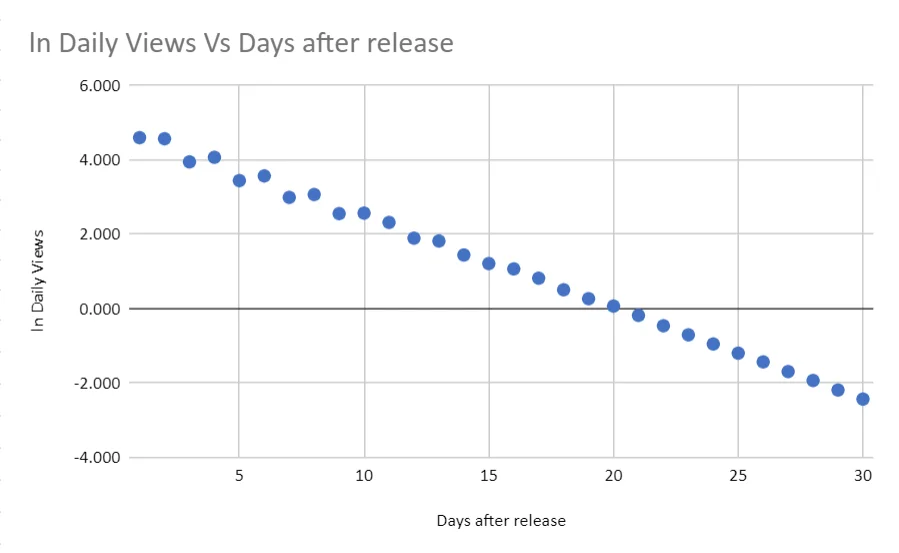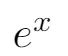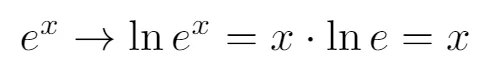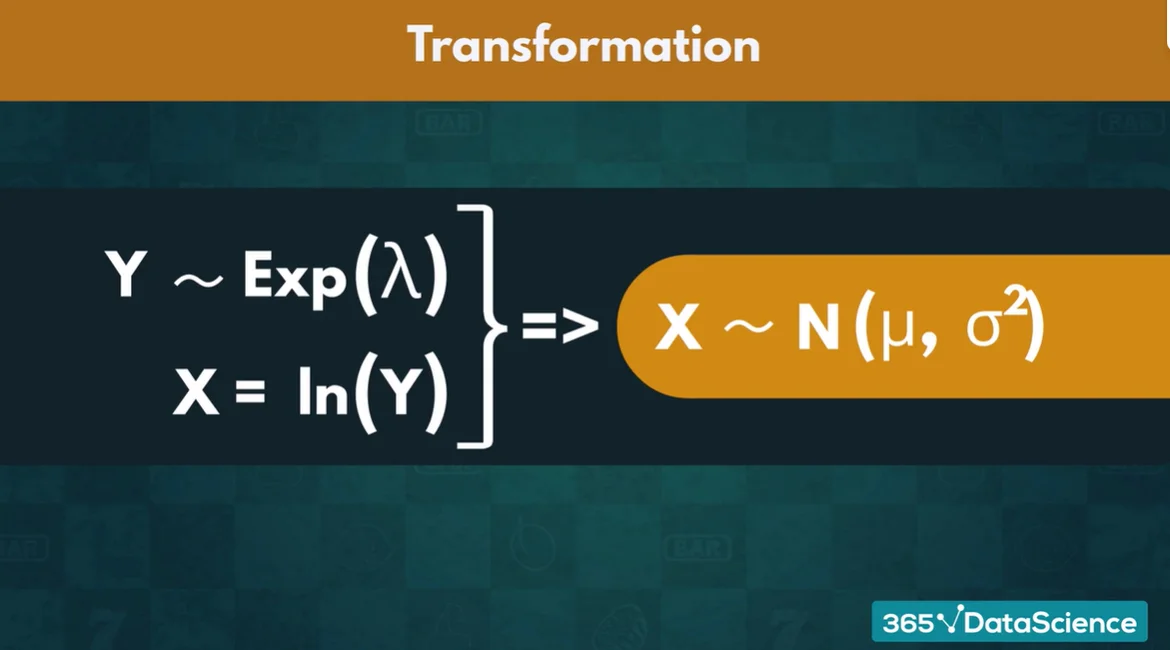09 Nov 2022

Posted on:

04 Jan 2022

1

# Transforming Exponential distribution to a Normal distribution.

Hi everyone,

I tried to transform the exponentially distributed data from you excel file "Daily Views.xlsx" to a Normal one by taking ln of the variable "Daily Views" but the resultant distrbution is completely different to a Normal distribution. Basically the result is the linearized distribution but not a normal one. Why is this? Is there another way to do this trasformation? Did I do something wrong?

Thanks.Instructor
Posted on:

05 Jan 2022

0

Hey Alberto,

An exponential function has the form (omitting any constants):By taking the natural logarithm of this function, you transform it as follows:The function y = x is a straight line. That is why the plot you get is transformed from an exponential to a line.

Unfortunately, I am not aware of a transformation that maps an exponential distribution to a normal one.

Hope this helps!

Kind regards,
365 Hristina

Posted on:

05 Jan 2022

1

Thanks a lot, Hristina.

I perfectly understand your explanation, threfore I would like to know if  the follwing picture gotten from the video explaining the exponential distribition is right or wrong. If I do not understand wrongly the picture shows that taking ln of a exponential distribution the resultant variable is normally distributed, but you just told me that this is not the case so could you please show me where am I getting lost?
Thanks, Alberto.Posted on:

09 Nov 2022

0

Hey! I was going to ask the same thing as Alberto. I think the statement:
Y ~ Exp(lambda) => X=ln(Y) ~ N(M, sigma^2) is incorrect.